# Chemistry: CBSE Sample Question Paper (2020-21) - 2 Notes | Study Chemistry Class 12 - NEET

## NEET: Chemistry: CBSE Sample Question Paper (2020-21) - 2 Notes | Study Chemistry Class 12 - NEET

The document Chemistry: CBSE Sample Question Paper (2020-21) - 2 Notes | Study Chemistry Class 12 - NEET is a part of the NEET Course Chemistry Class 12.
All you need of NEET at this link: NEET

Class - XII
Chemistry
TIME: 3 Hrs.
M.M: 80

General Instructions:
(a) There are 33 questions in this question paper. All questions are compulsory.
(b) Section A: Q. No. 1 to 16 are objective type questions. Q. No. 1 and 2 are passage based questions carrying 4 marks each while Q. No. 3 to 16 carry 1 mark each. MCQs or Reason Assertion Type based on given passage each carrying 1 mark.
(c) Section B: Q. No. 17 to 25 are Short Answer Questions and carry 2 marks each.
(d) Section C: Q. No. 26 to 30 are Short Answer Questions and carry 3 marks each.
(e) Section D: Q. No. 31 to 33 are Long Answer Questions carrying 5 marks each.(f) There is no overall choice. However, internal choices have been provided.
(g) Use of calculators and log tables is not permitted.

Section - A

Objective Type
Q.1.
The two monosaccharides are joined together by an oxide linkage formed by the loss of a water molecule. Such a linkage between two monosaccharide units through oxygen atom is called glycosidic linkage. In disaccharides, if the reducing groups of monosaccharides i.e., aldehydic or ketonic groups are bonded, these are non-reducing sugars, e.g., sucrose. On the other hand, sugars in which these functional groups are free, are called reducing sugars, for example, maltose and lactose.
A non reducing disaccharide ‘A’ on hydrolysis with dilute acid gives an equimolar mixture of D–(+)– glucose and D-(-)-Fructose.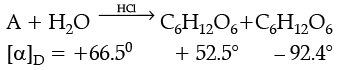(i) In the above reaction, reactant ‘A’ is
(a)
Glucose
(b) Sucrose
(c) Maltose
(d) Fructose
Ans. b
Solution. A–Sucrose (C12H22O11)

(ii) What is the mixture of D-(+) glucose and D-(+) fructose known as ?
(a)
Ano mers
(b) Racemic mixture
(c) Invert sugar
(d) Optical mixture
Ans. c
Solution. The mixture of D-(+)- glucose and D-(-)-Fructose is known as invert sugar.

(iii) Name the linkage that holds the two units in the disaccharide ?
(a)
(d) None of the above
Ans. b
Solution. The linkage which holds the two monosaccharide units through oxygen atom is called glycosidic linkage.

(iv) Glucose on reaction with acetic acid gives glucose pentaacetate. What does it suggest about the structure of glucose ?
(a)
C-1  is anomeric carbon
(b) C-5 is anomeric carbon
(c) 3’-O H groups are present
(d) 5’-OH groups are present
Ans. d
Solution. It indicates that 5’-OH groups are present which react with acetic acid to give glucose pentaacetate.

OR
Proteins are found to have two different types of secondary structures, viz. a-helix and b-pleated sheet structure. a-helix structure of protein is stabilised by

(a) Peptide bonds
(b) van der Waals forces
(c) H ydrogen bonds
(d) Dipole-dipole interactions
Ans. c
Solution. a-helix structure of protein is stabilised by hydrogen bonds. A polypeptide chain forms all possible hydrogen bonds by twisting into right-handed helix with the –NH group of each amino acid residue hydrogen bonded to >C=O of an adjacent turn of helix.

Q.2. Read the passage  given bellow and answer the following questions (1 x 4 = 4)
Scuba apparatus includes a tank of compressed air toted by the diver on his or her back, a hose for carrying air to a mouthpiece, a facemask that covers the eyes and nose, regulators that control air flow, and gauges that indicate depth and how much air remains in the tank. A diver who stays down too long, swims too deep, or comes up too fast can end up with a condition called “the bends.” In this case, bubbles of gas in the blood can cause intense pain, even death. In these following questions (Q. No. 12 - 16), a statement of assertion followed by a statement of reason is given. Choose the correct answer out of the following choices.
(a) Assertion and reason both are correct statements and reason is correct explanation for assertion.
(b) Assertion and reason both are correct statements but reason is not correct explanation for assertion.
(c) Assertion is correct statement but reason is wrong statement.
(d) Assertion is wrong statement but reason is correct statement.
(i) Assertion : Scuba divers may face a medical condition called ‘bends’.
Reason : ‘Bends’ can be explained with the help of Henry’s law as it links the partial pressure of gas to that of its mole fraction.
Ans. a
Solution. Henry’s law explains some biological phenomena like the ‘bends’ experienced by the scuba divers. Since mole fraction of a gas in the solution is a measure of its solubility.

(ii) Assertion : Bends is caused due to formation of nitrogen bubbles in the blood of scuba divers which blocks the capillaries.
Reason : Underwater high pressure increases solubility of gases in blood, while as pressure gradually decreases moving towards the surface, gases are released and nitrogen bubbles are formed in blood.
Ans. a
Solution. Scuba divers must cope with high concentrations of dissolved gases while breathing air at high pressure underwater. Increased pressure increases the solubility of atmospheric gases in blood. When the divers come towards surface, the pressure gradually decreases. This releases the dissolved gases and leads to the formation of bubbles of nitrogen in the blood. This blocks capillaries and creates a medical condition known as bends.

(iii) Assertion : Soft drinks and soda water bottles are sealed under high pressure.
Reason : High pressure maintains the taste and texture of the soft drinks.
Ans. c
Solution. The bottle is sealed under high pressure to increase the solubility of CO2 in soft drinks and soda water.

(iv) Assertion : Anoxia is a condition experienced by climbers which makes them suddenly agile and unable to think clearly.
Reason : At high altitudes the partial pressure of oxygen is less than that at the ground level.
Ans. d
Solution. At high altitudes the partial pressure of oxygen is less than that at the ground level. This leads to low concentrations of oxygen in the blood and tissues of people living at high altitudes or climbers. This leads to a condition called anoxia caused due to low oxygen in blood, making the climbers to become weak and unable to think clearly.

OR
Assertion : Solubility of gases in liquids decreases with rise in temperature.
Reason : As dissolution is an exothermic process, the solubility should decrease with increase of temperature.
Ans. a
Solution. Solubility of gases in liquids decreases with rise in temperature. As dissolution is an exothermic process, the solubility should decrease with increase of temperature.

Following questions (No. 3-11) are Multiple Choice Questions carrying 1 mark each:
Q.3. KMnO4 acts as an oxidizing agent in the acidic medium. The number of moles of KMnO4 that will be needed to react with one mole of sulphide ion in acidic solution is:
(a)
2/5
(b) 3/5
(c) 4/5
(d) 1/5
Ans. a
Solution. 2MnO4 + 5S2– + 16H+ → 2Mn2+ + 5S + 8H2O For 5 moles of S the number of moles of
KMnO4 = 2
For 1 mole of S the number of moles of KMnO4 = 2/5

Q.4. The correct increasing order of basic strength for the following compounds is
__________.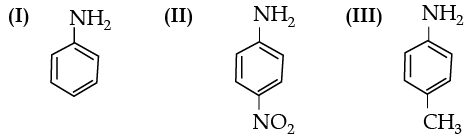(a) II<III< I
(b) III < I < II
(c) III < II < I
(d) II < I < III
Ans. d
Solution.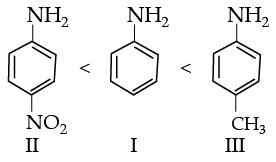Electron withdrawing group decreases the basic strength while electron releasing groups increases the basic strength of aniline.

OR

The best reagent for converting 2–phenylpropanamide into 2-phenylpropanamine is _____.
(a)
excess H2
(b) Br2 in aqueous NaOH
(c) Iodine in the presence of red phosphorus
(d) LiAlH4 in ether
Ans. d
Solution.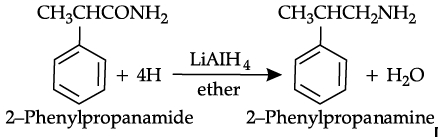Q.5. The best reagent for converting, 2-phenylpropanamide into 1-phenylethanamine is ____.
(a)
excess H2/Pt
(b) NaOH/Br2
(c) NaBH4/methanol
(d) LiAlH4/ether
Ans. b
Solution.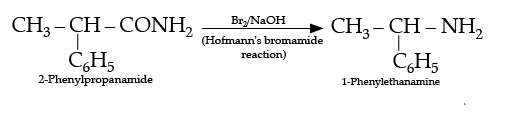OR
Aniline reacts with sodium nitrite and hydrochloric acid at 273-278K to give.
(a)
Benzene
(b) Benzenediazonium chloride
(c) Chlorobenzene
(d) o-chloroaniline
Ans. b
Solution.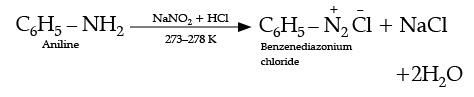Q.6.The IUPAC name of the compound shown below is: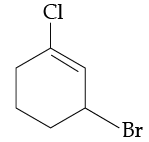(a)
2-bromo-6-chlorocyclohex-1-ene
(b) 6-bromo-2-chlorocyclohexene
(c) 3-bromo-1-chlorocyclohexene
(d) 1-bromo-3-chlorocyclohexene
Ans. c

OR
Identify the structure of 1-bromo-4-chlorobut-2-ene.
(a)
CH3CH=CBrCH2Cl
(b) BrCH=CHCH2CH2Cl
(c) CH3CHBrCH=CHCl
(d) BrCH2CH=CHCH2Cl
Ans. d

Q.7. Which alkyl halide from the following pair is chiral and undergoes faster SN2 reaction ?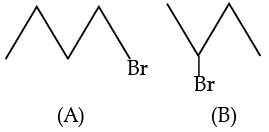(a)
A is chiral and B undergoes faster SN2 reaction
(b) A is chiral and undergoes faster SN2 reaction
(c) B is chiral and undergoes faster SN2 reaction
(d) B is chiral and A undergoes faster SN2 reaction
Ans. d

Q.8. Which of the following process is responsible for the formation of delta at a place where rivers meet the sea?
(a)
Emulsification
(b) Colloid formation
(c) Coagulation
(d) Peptization
Ans. c
Solution. A delta is formed at a place where rivers meet the sea due to the process of setting down of colloidal particles. The ions which are present in sea water are responsible for coagulation.

Q.9. Write the IUPAC name of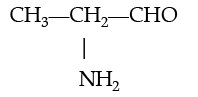(a)
1-Aminopropanaldehyde
(b) 2-Aminopropanal
(c) 1-Aminoethan-1-al
(d) None of the above
Ans. b

OR
Identify the structure of an isomer of compound C4H9Br which is most reactive towards SN1 reaction.
(a)
2-bromo-1-methylpropane
(b) 2-methyl-bromopropane
(c) 2-bromo-2-methylpropane
(d) 2-methyl-2-bromopropane
Ans. c
Solution.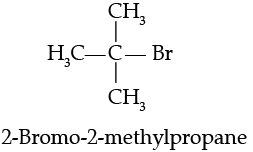Q.10. Which of the following pairs represents anomers?
(a)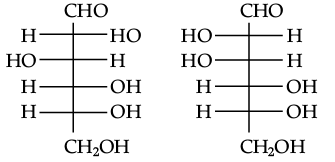(b)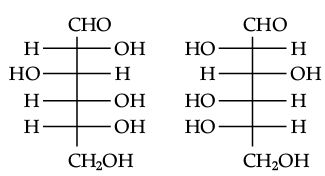(c)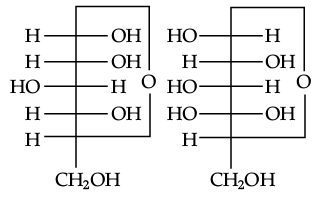(d)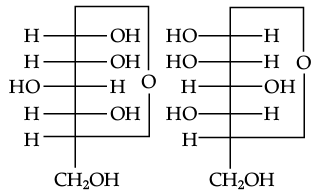Ans. c
Solution. The isomers, which differ only in the configuration of the hydroxyl group at C-1, are called anomers and are referred to as a and β-forms.

Q.11. Which of the following is not tetrahedral in shape?
(a)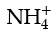(b) SiCl4
(c) SF4
(d)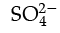Ans. c

In the following questions (Q. No. 12 - 16) a statement of assertion followed by a statement of reason is given. Choose the correct answer out of the following choices.
(A)
Assertion and reason both are correct statements and reason is correct explanation for assertion.
(B) Assertion and reason both are correct statements but reason is not correct explanation for assertion.
(C) Assertion is correct statement but reason is wrong statement.
(D) Assertion is wrong statement but reason is correct statement.
Q.12. Assertion (A) : When NaCl is added to water a depression in freezing point is observed.
Reason (R) : The lowering of vapour pressure of a solution causes depression in the freezing point.
Ans. a
Solution. When a non-volatile solute is added to water, freezing point lowers due to lowering of vapour pressure.

Q.13. Assertion (A) : Cu2+ iodide is not known.
Reason (R) : Cu2+ oxidises I to iodine.
Ans. a
Solution. Cu2+ oxidises iodide to iodine hence cupric iodide is converted to cuprous iodide.

Q.14. Assertion (A) : Nickel can be purified by Mond's process.
Reason (R) : Ni(CO)4 is a volatile compound which decomposes at 460 K to give pure Ni.
Ans. a
Solution. When nickel (Ni) is treated with carbon monoxide it forms nickel tetracarbonyl Ni(CO)4, while impurities are left behind. When the vapour of Ni(CO)4 is heated at 460 K, it is decomposed to give pure nickel while carbon monoxide is removed as gas.

Q.15. Assertion (A) : Colloidal solutions do not show Brownian motion.
Reason (R) : Brownian motion is responsible for stability of sols.
Ans. d
Solution. Colloidal particles show Brownian movement and it is responsible for the stability of colloidal solution.

Q.16. Assertion (A) : Colloids are stable.
Reason (R) : Brownian movement has a stirring effect, which does not allow the particles to settle.
Ans. a
Solution. Colloids are stable due to Brownian movement which is the movement of colloidal particles striking against the dispersion medium. It prevents them from settling down.

Section - B

The following questions (Q.No 17 – 25) are Short Answer Type-I and carry 2 marks each.
Q.17. Explain why on addition of 1 mol of NaCl to 1 litre of water, the boiling point of water increases, while addition of 1 mol of methyl alcohol to one litre of water decreases its boiling point.
Ans. Sodium chloride (NaCl) is a non-volatile solute, therefore, addition of NaCl to water lowers the vapour pressure of water. As a result, boiling point of water increases. Methyl alcohol, on the other hand, is more volatile than water, hence, its addition increases the total vapour pressure of the solution and in decrease in boiling point of water results.

Q.18. Explain why on addition of 1 mol glucose to 1 litre water the boiling point of water increases.
Ans. Vapour pressure of the solvent decreases in the presence of non-volatile solute (glucose) as the mole fraction of water decreases hence boiling point increases.
OR
Why a mixture of carbon disulphide and acetone shows positive deviation from Raoult’s law ? What type of azeotrope is formed by this mixture?

Ans. Intermolecular forces of attraction between carbon disulphide and acetone are weaker than the pure components.
Minimum boiling azeotrope at a specific composition.

Q.19. Account for the following:
(i) The two oxygen-oxygen bond lengths in ozone molecule are identical.
(ii) Most of the reactions of fluorine are exothermic.
Ans.
(i) Due to resonance the two O-O bond lengths are identical.
(ii) Due to strong bond formed by it with other elements.

(i) In ozone, the central oxygen atom forms one single bond with a terminal oxygen atom and double bond with other terminal oxygen atom. The π electrons of double bond are delocalised over the three oxygen atoms. This results into resonance hybrids with the average bond distance of the single and double bond.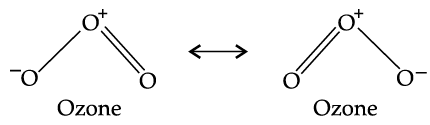Q.20. Which of the following solutions has higher freezing point ? 0.05 M Al2(SO4)3, 0.1 M K3[Fe(CN)6] Justify.
Ans.

0.05 M Al2(SO4)3 has higher freezing point.
0.05 M Al2(SO4)3 : i = 5,
ΔTf ∝ No. of particles;
ΔTf = i × concentration
= 5 × 0.05

= 0.25  moles of ions
0.1 M K3[Fe(CN)6] : i = 4,
= 4 x 0.1
= 0.4 moles of ions

OR
Calculate the molality of ethanol solution in which the mole fraction of water is 0.88.
Ans. Mole fraction of water, XH2O = 0.88
Mole fraction of ethanol, XC2H5OH =  1 – 0.88
= 0.12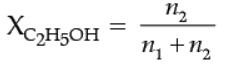n2 = number of moles of ethanol.
n1 = number of moles of water.
Molality of ethanol means the number of moles of ethanol present in 1000 g of water.
n1 = 1000/18 = 55.5 moles
Substituting the value of n1 in equation (1)

n2/55.5 + n= 0.12
n2 = 7.57 moles
Molality of ethanol (C2H5OH) = 7.57 m
Alternatively,
Mole fraction of water = 0.88
Mole fraction of ethanol = 1 – 0.88 = 0.12
Therefore 0.12 moles of ethanol are present in 0.88 moles of water.

Mass of water = 0.88 × 18 =15.84 g of water.

Molality = number of moles of solute (ethanol) present in 1000 g of solvent (water)
= 0.12 × 1000 / 15.84 = 7.57 m
Molality of ethanol (C2H5OH) = 7.57 m

Q.21. What is an anomer? Structure of a disaccharide formed by glucose and fructose is given below. Identify anomeric carbon atoms in monosaccharide units.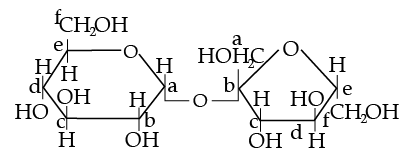Ans.

‘a’ carbon of glucose and ‘b’ carbon of fructose are the anomeric carbon atoms in the monosaccharide units. The isomers which differ only in the configuration of the hydroxyl group at C-1 are called anomers and are referred to as α- and β-forms.

OR

Define the following terms:
(ii) Invert sugar
Ans.

(i) Glycosidic linkage: The two monosaccharides units are joined together through an oxide linkage formed by loss of a molecule of H2O.
(ii) Invert sugar: Hydrolysis of sucrose brings about a change in sign of rotation from dextro (+) to laevo (–) and the product is known as invert sugar.

Q.22. (i) Why phenol is more acidic than ethanol?
(ii)Write the mechanism of acid dehydration of ethanol to yield ether: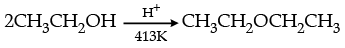Ans.
(i) Due to resonance, phenoxide ion is more stable than phenol whereas there is no resonance is alkoxide ion / explained with the help of resonating structures.
(ii) Mechanism of acid dehydration of ethanol: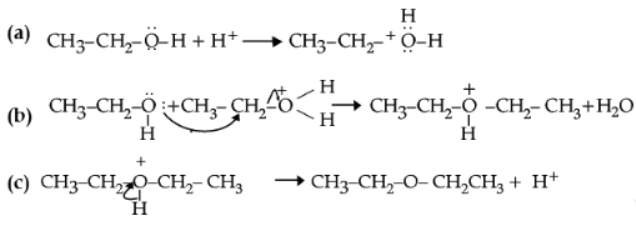OR
(i) Write Reimer-Tiemann reaction.
(ii) Write the mechanism of acid dehydration of ethanol to yield ethene: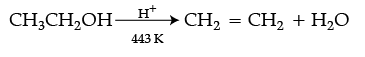Ans.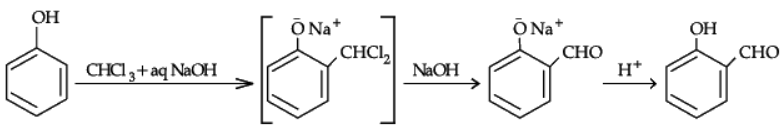(ii) Step 1: Formation of protonated alcohol.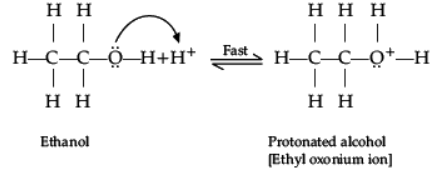Step 2: Formation of carbocation: It is the slowest step and hence, the rate determining step of the reaction.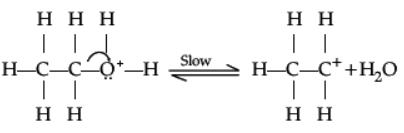Step 3: Formation of ethene by elimination of a proton.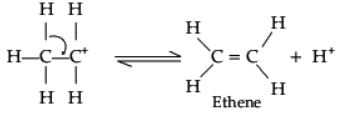Q.23. Write the reagents required in the following reactions:
(i) CH2 = CH – CH2 OH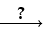CH2 = CH – CHO
(ii) CH3 – COOHCH3 – CONH2
Ans. (i) PCC/Cu at 573 K
(ii) NH3, D(heat).

OR
(i) Arrange the following compounds in the increasing order of their acid strength: p-cresol, p-nitrophenol, phenol
(ii) Write the mechanism (using curved arrow notation) of the following reaction;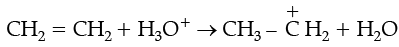Ans.
(i)
C6H5COCH3<CH3COCH3<CH3CHO.
(ii) CH3COOH<Cl – CH2 – COOH<F – CH2 – COOH

Q.24. Give reasons: (i) C – Cl bond length in chlorobenzene is shorter than C – Cl bond length in CH3 – Cl.
(ii) The dipole moment of chlorobenzene is lower than that of cyclohexyl chloride.
Ans.
(i) Hoffmann-bromamide degradation reaction: When an amide is treated with bromine in aqueous or ethanolic solution of sodium hydroxide, a primary amine with one carbon atom less than the original amide is produced. This degradation is known as Hoffmann bromamide degradation reaction.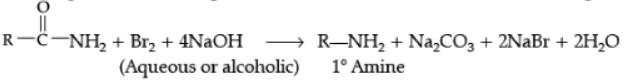Example,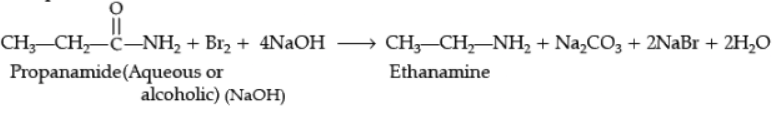(ii) Carbylamine reaction: It is used as a test for detection of primary amines. When aliphatic or aromatic primary amines are heated with chloroform and alcoholic potassium hydroxide, carbylamine or isocyanides having foul smell are formed. Secondary and tertiary amines do not respond to this test.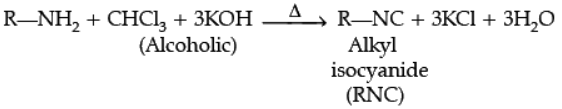Q.25. (i) Allyl chloride can be distinguished from vinyl chloride by NaOH and silver nitrate test. Comment.
(ii) Alkyl halide reacts with lithium aluminium hydride to give alkane. Name the attacking reagent which will bring out this change.
Ans.
(i) Vinyl chloride does not respond to NaOH and silver nitrate test because of partial double bond character due to resonance.

(ii) Hydride ion/H

OR
Following compounds are given to you : 2-Bromopentane, 2-Bromo-2-methylbutane, 1-Bromopentane
(i) Write the compound which is most reactive towards SN2 reaction.
(ii) Write the compound which is optically active.
(iii) Write the compound which is most reactive towards b-elimination reaction.
Ans.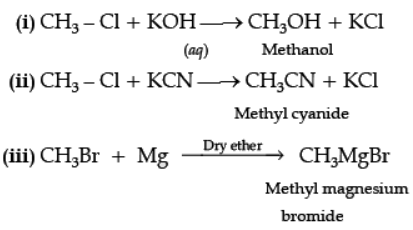Section - C

Q.No 26 -30 are Short Answer Type-II carrying 3 marks each.
Q.26. Give reasons for the following:
(i) (CH3)3 P = O exists but (CH3)3 N = O does not.
(ii) Oxygen has less electron gain enthalpy with negative sign than sulphur.
(iii) H3PO2 is a stronger reducing agent than H3PO3.
Ans.
(i) Because as N can't form 5 covalent bonds as its maximum covalency is three. The octet cannot be extended as it doesn't have d orbital, while P can extend its octet as it has empty d orbital.
(ii) This is due to very small size of oxygen atom, repulsion between electrons is large in relatively small 2p sub-shell.
(iii) In H3PO2 there are 2P – H bonds, whereas in H3PO3 there is 1 P-H bond.

OR
(i) What happens when:
(a) Chlorine gas reacts with cold and dilute solution of NaOH?
(b) XeF2 undergoes hydrolysis?
(ii) Assign suitable reason for the following:
Out of noble gases only Xenon is known to form established chemical compounds.
Ans.
(i) F2 has lower bond dissociation energy than Cl2 because the size of F2 is much smaller than Cl2 as a result interelectronic repulsion works and make F2 weak.
(ii) Helium is used in filling balloons for meteorological observations.
(iii) XeF2 + PF5 ® [XeF]+ [PF6]

Q.27. What happens when:
(i)
CH3–Cl is treated with aqueous KOH ?
(ii) CH3–Cl is treated with KCN ?
(iii) CH3–Br is treated with Mg in the presence of dry ether?
Ans.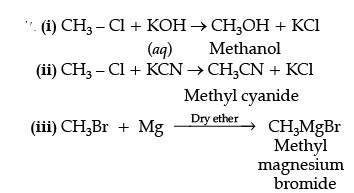Q.28. (i) What type of aldehydes undergo Cannizzaro reaction?
(ii)
Arrange the following compounds in increasing order of their property as indicated:
(a) CH3COCH3, C6H5COCH3, CH3CHO (reactivity towards nucleophilic addition reaction)
(b) Cl – CH2 – COOH, F – CH2 – COOH, CH3 – COOH (acidic character)
Ans. (i) Having no α - hydrogen
(ii) (a) C6H5COCH3 < CH3COCH3 < CH3CHO
(b) CH3COOH < Cl – CH2 – COOH < F – CH2 –COOH

Q.29. Give reason for the following:
(a)
Compounds of transition elements are generally coloured.
(b) MnO is basic while Mn2O7 is acidic.
(c) Many of the transition elements are known to form interstitial compounds.
Ans. (a) Due to d-d-transition.
(b) Due to higher oxidation state of Mn2O7/Due to high polarizing power of Mn(VII).
(c) In the crystal lattice, transition elements have interstitial vacant spaces into which small sized non-metal atoms such as H, B, C or N are trapped. These compounds are non-stoichiometric, neither typically ionic nor covalent. e.g., TiC, MH, Fe3H etc.

Q.30. An element crystallizes in fcc lattice with cell edge of 400 pm. Calculate its density if 250 g of this element contain 2.5 × 1024 atoms.
Ans. In fc c, z = 4;
d = (z × M)/a3 × NA (i)
No. of atoms = w/M x NA
2.5 x 1024 = 250 g/M × NA
M = [250 × NA]/2.5 × 1024 (ii)
Putting values of M in equation (i)
d = 4 × 250 g × NA /[2.5 × 1024 atoms × (400 × 10-10 cm3) × NA]
d = 6.25 g/cm3    (or any other correct method)

OR
An element exists in bcc lattice with a cell edge of 288 pm. Calculate its molar mass if its density is 7.2 g/cm3.
Ans.
In bcc, z = 2;
d = (z × M)/a3 × NA    (i)
Putting values of M in equation (i)
M = 7.2g/cm3 × (288 × 10-10 cm)3 NA/2 
= 51.8 g/mol    (or any other correct method)

a = 288 pm = 288 × 10-10 cm
d = 7.2 g/cm3
In bcc, z = 2   NA = 6.022 × 1023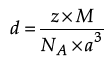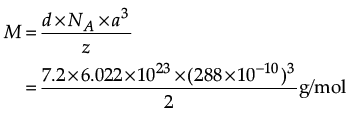= 51.7869 g/mol
≈ 51.8 g/mol

Section - D

Q.No 31 to 33 are Long Answer Type carrying 5 marks each.
Q.31. Write the hybridization and magnetic character of the following complexes:
(i)
[Fe(H2O)6]2+
(ii) [Fe(CO)5]
Ans.
(i) sp3d2, Paramagnetic
(ii) sp3d2, Paramagnetic
(i) In [Fe(H2O)6]2+the oxidation state of Fe is +2. The outermost electronic configuration of Fe is 3d64s24p0. H2O is weak field ligand and do not cause pairing up of electrons.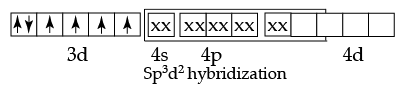In this complex, there are 4 unpaired electrons. Therefore, it involves sp3d2  hybridisation and is paramagnetic.

(ii) In [Fe(CO)5] the oxidation state of Fe is zero. The outermost electronic configuration of Fe is 3d64s24p0. CO is strong field ligand, causes pairing up of 4s electrons into the 3d orbitals.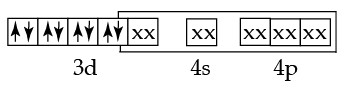In this complex, there is no unpaired electron. Therefore, it involves dsp3 hybridisation and is diamagnetic.

OR
Write down the IUPAC name for each of the following complexes and indicate the oxidation state, electronic configuration and coordination number. Also give stereochemistry and magnetic moment of the complex:

(a) K[Cr(H2O)2(C2O4)2].3H2O
(b) [Co(NH3)5Cl]Cl2
(c) [CrCl3(py)3]
(d) Cs[FeCl4]
(e) K4[Mn(CN)6]
Ans. (a)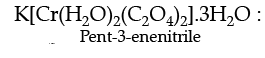Oxidation state of chromium: 3
Electronic configuration: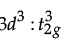Coordination number : 6
Shape: Octahedral
Stereochemistry: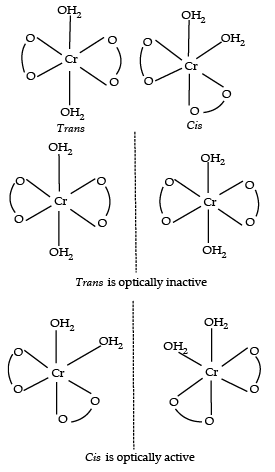Magnetic moment: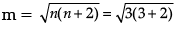= √15 = 3.872 ≈ 4 BM

(b) [Co(NH3)5Cl]Cl2 :
IUPAC name :
Pentaamminechloridocobalt(III)chloride
Oxidation state of Co : +3
Coordination number : 6
Shape : Octahedral
Electronic configuration : d6 : t2g6
Stereochemistry :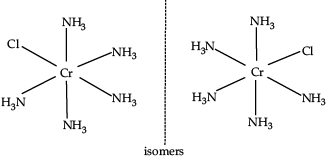Magnetic moment = 0
(c) CrCl3(py)3 :
IUPAC name: Trichloridotripyridinechromium (III)
Oxidation state of chromium : +3
Electronic configuration : d3 : t2g3
Coordination number : 6
Shape : Octahedral
Stereochemistry :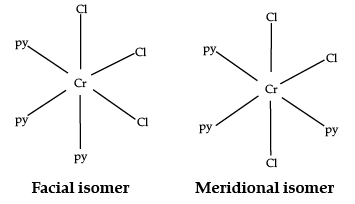Both isomers are optically active. Therefore, a total of four isomers exist.

Magnetic moment :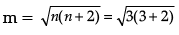= √15 = 3.872 ≈ 4 BM

(d) Cs[FeCl4] :
IUPAC name :
Caesiumtetrachloroferrate (III)
Oxidation state of Fe : +3
Electronic configuration : d6 : eg2t2g3
Coordination number : 4
Shape : Tetrahedral
Stereochemistry : Optically inactive
Magnetic moment :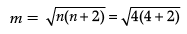= √24 = 4.89 ≈ 5 BM

(e) K4[Mn(CN)6] :
IUPAC name :
Potassium hexacyanomanganate(II)
Oxidation state of manganese : +2
Electronic configuration : d5+ : t2g5
Coordination number : 6
Shape : Octahedral.

Stereochemistry : Optically inactive
Magnetic moment :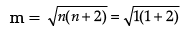= √3 =1.732BM

Q.32. (i) Explain how does the –OH group attached to a carbon of benzene ring activate it towards electrophilic substitution ?
(ii) Following compounds are given to you : 2-Bromopentane, 2-Bromo-2-methylbutane, 1-Bromopentane
(a) Write the compound which is most reactive towards SN2 reaction.
(b) Write the compound which is optically active.
(c) Write the compound which is most reactive towards b-elimination reaction.

Ans. (i) The –OH group is an electron-donating group. Thus, it increases the electron density in the benzene ring as shown in the given resonance structure of phenol.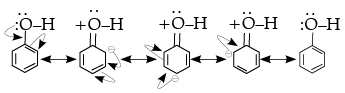As a result, the benzene ring is activated towards electrophilic substitution.
(ii) (a) 1-Bromopentane
(b) 2-Bromopentane
(c) 2-Bromo-2-methylbutane

OR
(i) Ortho and para nitrophenols are more acidic than phenol. Draw the resonance structures of the corresponding phenoxide ions.
(ii) Arrange the following compounds in increasing order of acidity and give a suitable explanation.
Phenol, o-nitrophenol, o-cresol.
Ans.
(i) Resonance structure of the phenoxide ion :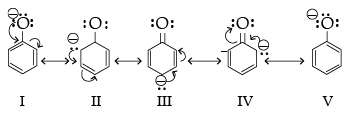Resonance structures of ortho-nitrophenoxide ion : are formed by the loss of proton from ortho-nitrophenol.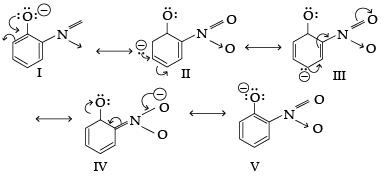Resonance structures of para-nitrophenoxide ion :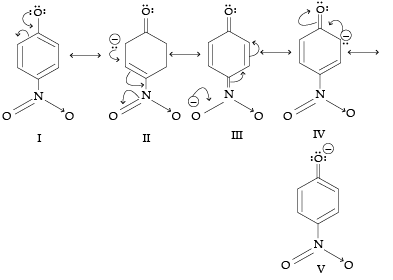It can be observed that the presence of nitro groups increases the stability of ortho- and para-nitrophenoxide ions in comparison to phenoxide ions. Hence, ortho and para nitrophenols are more acidic than phenol.

(ii) Increasing order of acidity : o-cresol < phenol < o-nitrophenol.
In cresol, the electron releasing group (–CH3) gives electrons and intensify the charge on phenoxide ion and therefore makes it unstable. Therefore, o-cresol is less acidic than phenol. In o-nitrophenol, the electron withdrawing (–NO2) group withdraws electrons and disperses the negative charge and stabilizes the phenoxide ion. Therefore, o-nitrophenol is more acidic than phenol.

Q.33. Write the structure of A, B, C, D and E in the following reactions :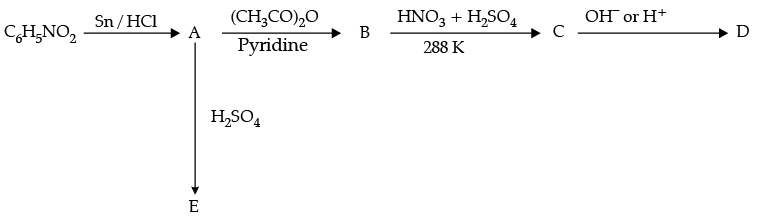Ans.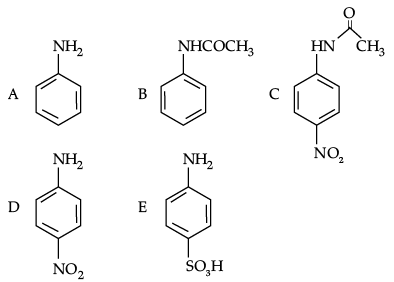OR
Predict the reagent or the product in the following reaction sequence: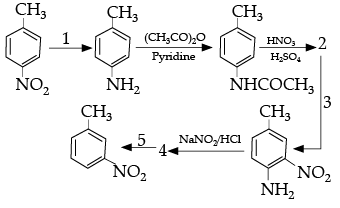Ans.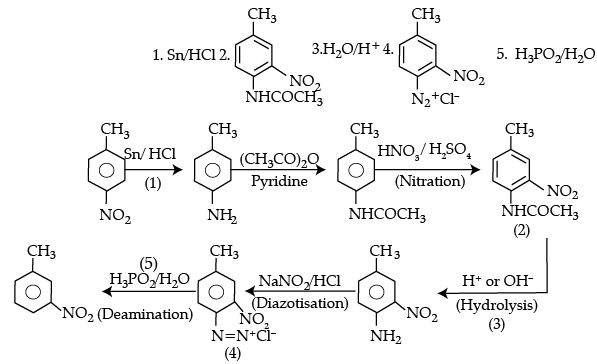The document Chemistry: CBSE Sample Question Paper (2020-21) - 2 Notes | Study Chemistry Class 12 - NEET is a part of the NEET Course Chemistry Class 12.
All you need of NEET at this link: NEETUse Code STAYHOME200 and get INR 200 additional OFF

## Chemistry Class 12

110 videos|306 docs|259 tests

### How to Prepare for NEET

Read our guide to prepare for NEET which is created by Toppers & the best Teachers

Track your progress, build streaks, highlight & save important lessons and more!

,

,

,

,

,

,

,

,

,

,

,

,

,

,

,

,

,

,

,

,

,

;